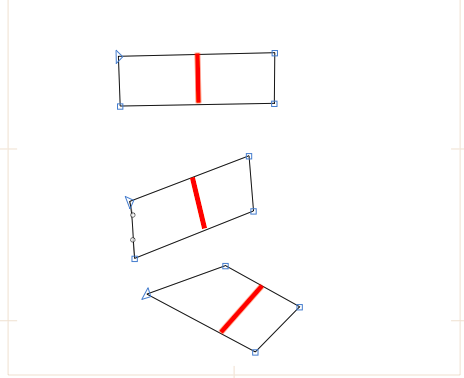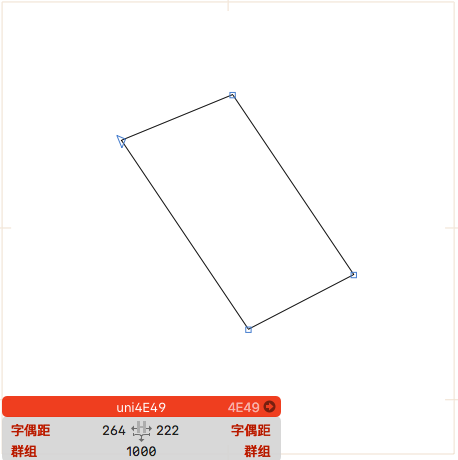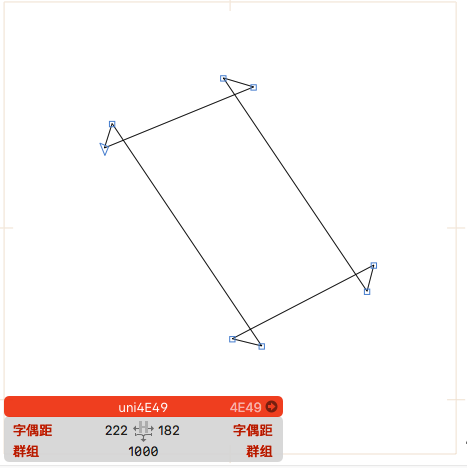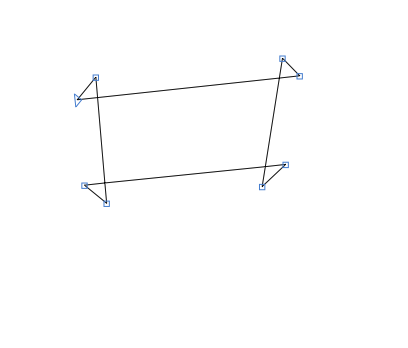# Can I use Python scripts to control and adjust the distance between any two parallel lines

Can I use Python scripts to control and adjust the distance between any two parallel lines?As shown in the following figure, the red line segment represents the distance between two parallel lines.Yes.

It would be tricky to determine which parallel line you mean – in your screenshot the top stroke could also have the left and right line as parallel lines.

Either you use a form of user input (selecting two segments) or rely on determining the longest two segments that are parallel. Up to you.

Then either use open corners to move the respective segment orthogonally to its orientation (lazy method) or directly calculate the new intersection points with the adjoining segments (big brain method).

Thank you very much. Can you provide a code reference

For which approach? I’m afraid you’ll have to decide on how exactly you want this to work. Please consider the options/questions I proposed in my previous replyi want to rely on determining the longest two segments that are parallel , and then use open corners to move the respective segment orthogonally to its orientation (lazy method) ,thnaks very much!!

I’ll get back to you on this tomorrow, if nobody else does. Please remind me otherwise.

thanks very much

Hello, here’s a start:

``````from math import degrees, atan2

tolerance = 1 # define the maximum angle that the segments can deviate by (useful for non-orthogonal segments)
path = Layer.paths

def get_segment_angle(segment):
nodes = segment.objects()
x_diff = nodes.x - nodes.x
y_diff = nodes.y - nodes.y
angle = abs(degrees(atan2(abs(y_diff), abs(x_diff))))
return(angle)

segments_by_length = sorted(path.segments, key=lambda segment: segment.length())  # sorts the segments of the path into a list by their length

angle_diff = abs(get_segment_angle(segments_by_length[-1]) - get_segment_angle(segments_by_length[-2]))  # calculates the deviation in angle between the segments

if angle_diff <= tolerance:
for node in segments_by_length[-1].objects():  # objects() returns the two nodes that consitute the start and end of the segment
Layer.openCornerAtNode_offset_(node, 100)  # open the corner at each end
for node in segments_by_length[-2].objects():
Layer.openCornerAtNode_offset_(node, 100)
``````

This will find the two longest segments in the first path of your current layer. It then checks if these two segments are (almost) parallel. If they are, it opens the corners of the two segments.

I would kindly request someone much smarter than me to explain the maths of how to move a node along a line (in order to skip the step of opening corners).

If the code is unclear, please ask. I don’t have the time right now to code moving the segments apart, sorry.

Why? Just get the two segments and move them at the ‘red line’ angle, no?

No, because this would change the angle of the adjacent segments, that’s the tricky part. Which is why I used the open corner workaround.

That probably depends on how @mrsjing meant to use it and what these lines are attached to

Thanks，The function of this part of code is to open the angle between two line segments, right. The rest is how to move in the vertical direction, right？The following figure shows the effect of executing the code.These functions, such as degrees and atan2, cannot be found in the official Glyphs.app Python Scripting API Documentation document. Where can I learn their functions and related knowledge.

Where can I learn the relevant knowledge of moving the relevant segment orthogonally to its orientation? I cannot find inspiration in the official documentation

`degrees` and `atan2` are common graphics function and not specific to Glyphs. In Python, they are provided by the `math` module (hence the `from math import degrees, atan2` on the first line).

I am not 100% sure what you are trying to do but this code might help:

After writing the code, which parameter controls the translation distance, such as the translation distance of 100

``````import math
def movePoint(x1, y1, x2, y2, t, offset):
angle = math.atan2(y2 - y1, x2 - x1)
print(angle)
tx = x1 + (x2 - x1) * t
ty = y1 + (y2 - y1) * t
dx = math.sin(angle) * offset
dy = math.cos(angle) * offset
print(NSMakePoint(tx + dx, ty - dy))
return NSMakePoint(tx + dx, ty - dy)
for thisPath in Layer.paths:
print(len(thisPath.nodes))
P1 = thisPath.nodes
print(P1)
P2 = thisPath.nodes
print(P2)
position = movePoint(P1.x, P1.y, P2.x, P2.y, 1, 10)
print(position)
``````

Thanks,but executing the above code, the line segment did not moveCan you help me? Because I’m a novice and the rest of the code is difficult to complete, do I need to move along the open corners

Can you help me complete the code? As a novice, I really cannot complete it

Can you help me complete the remaining code? I really don’t know how to write it